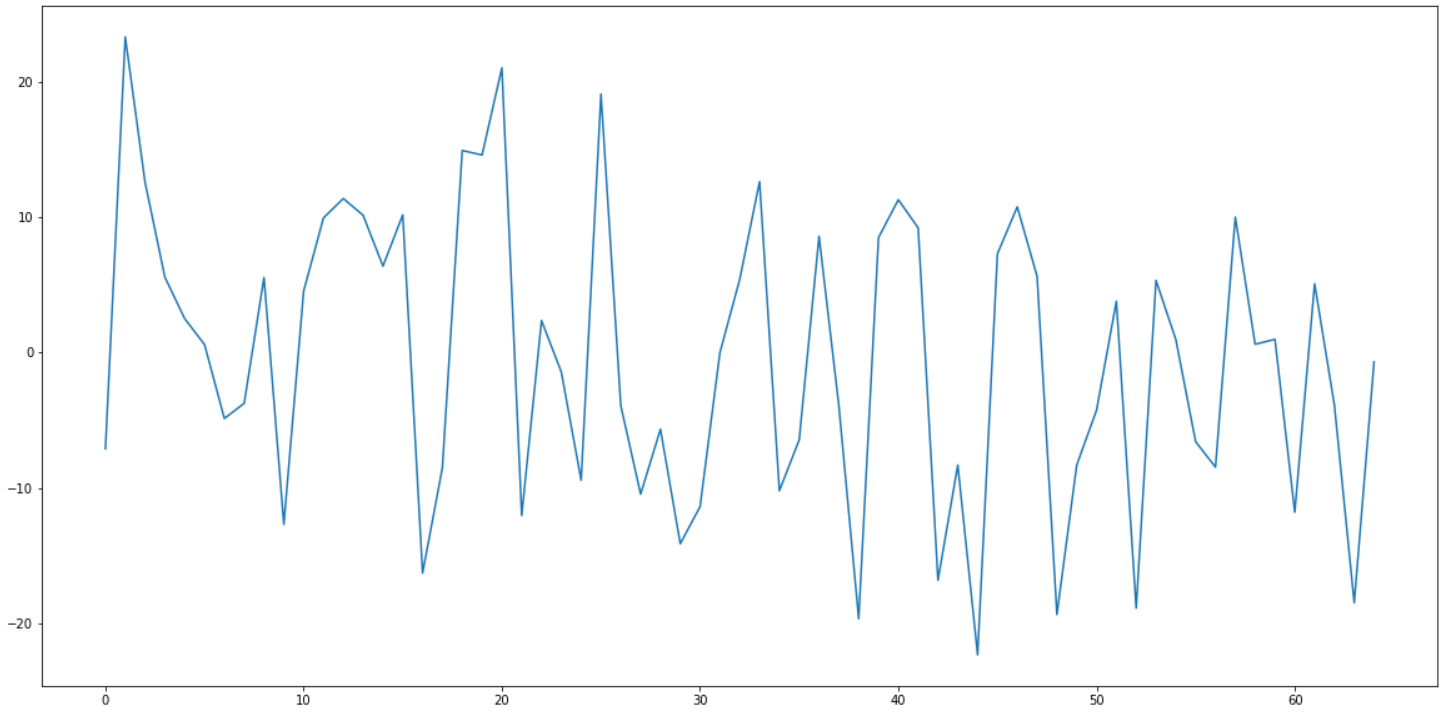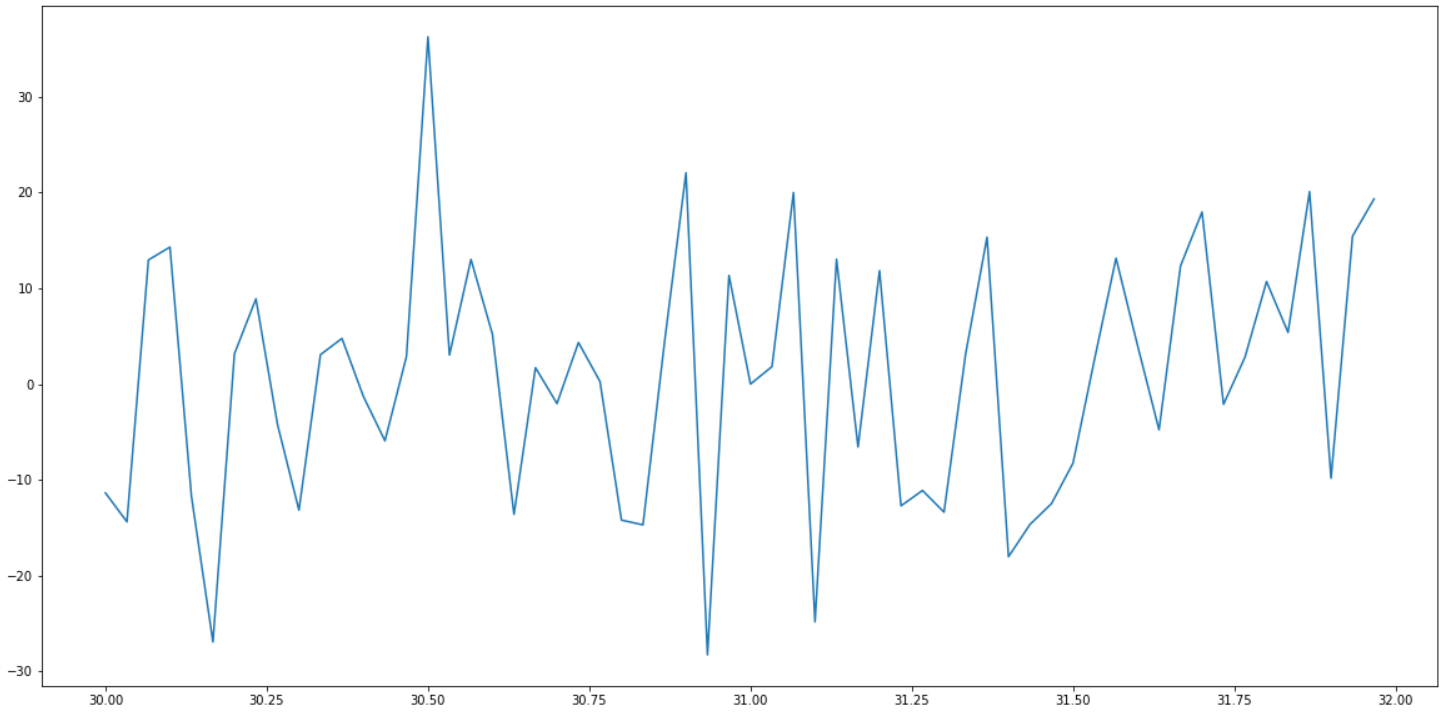# Nevermind XOR - Deep Learning has an issue with Sin

More precisely, even the best neural networks can not be trained to approximate periodic functions using stohastic gradient descent. (empirically, prove me wrong!)

# Simulating a clock

The problem that led me into this sinkhole is an attempt to model biological rhythms. People sleep every 24 hours, the nature has a distinct 365 day cycle, and over a month the Moon goes from new to full and back. I wanted to capture that repetition relative to the current instant in time, which led to the Clock Problem:

Given the number of seconds since some moment T0 defined to be 0 days 0:00:00, train a neural network to approximate the numbers you would see on a digital clock. For example, `-1` (second) would be `23:59:59`.

Expecting that to be a dead simple task, I built an infinite dataset, that would sample a random instant in time from a period of 120 years, and fed it into a SIREN - neural network with sinusoidal activations.

To my surprise, despite playing with its frequency scale hyperparameter, the network, essentially, never converged.

I tried to use regular MLP with GELU activations, and got approximately the same result.

Research on the topic only brought Neural Networks Fail to Learn Periodic Functions and How to Fix It, which, as you might have guessed it, did not really work on the Clock Problem. Their `x + sin(x) ** 2` activation only really worked when the number of full cycles in the dataset was less than the number of paramters of the network, which completely misses the point.

# Simplifying the problem

You can quickly see how inappropriate gradient descent is for the problem if we just simplify it a little. Let’s try approximating this trivial function:

``````FREQUENCY_SCALE = 31

def func(x):
``````

There is a a PyTorch module, that surely should solve the problem:

``````class Sin(torch.nn.Module):
def __init__(self):
super().__init__()
self.freq = torch.nn.Parameter(torch.randn(1))

def forward(self, x):
``````

Here, we only need to find the frequency, and the module will match our target function exactly! Let’s try it out:

``````net = Sin()
opt = torch.optim.SGD(net.parameters(), lr=0.0001)

BATCH_SIZE = 32

for batch_idx in range(1000*1000):

batch = (torch.rand(size=[BATCH_SIZE, 1], device=device) * 2 - 1) * 1000

out = net(batch)
expected = func(batch)

loss = ((out - expected) ** 2).mean()

loss.backward()

opt.step()

if batch_idx % 1000 == 0:
print(f'{loss.detach().cpu().item()}')
``````

If you run this on your machine, you will see something like this:

0.775499165058136 1.3729740381240845 1.0878400802612305 0.7583212852478027 1.3061308860778809 0.6976296305656433 1.0671122074127197 0.9739978909492493 0.947789192199707

The loss just floats around 1 and never converges.

But we actually know the answer! Just insert this line:

``````net.freq.data = torch.tensor(, dtype=torch.float32)
``````

and your loss will turn to zero instantly:

0.0 0.0 0.0 0.0

# Why can’t we train the `sin`?

For the given `x0` the derivative of our scaled `sin` with respect to `freq` parameter is `x0 * cos(x0 * freq)`. There are two things to note:

1. The scale of the derivative value depends on how far x0 is from 0. This is bad, as really that dependency only makes sense within `[-x0/freq*pi, +x0/freq*pi]` interval.
2. The derivative does not really point where we want to go. Instead, its direction only depends on whether the `x0 * freq` is closer to `2n*pi` or `(2n+1)*pi` for some `n`. And that value will vary wildly for different samples in the batch.

This is how the gradient of the `freq` paramter looks like on a large random batch of points:Can you spot the 0 at 31? As you can see, around it the gradient is all over the place.

Even in the immediate vicinity of 31, it does not behave well:# What to do about it?

I don’t know. Perhaps a form of Fourier transform or a wavelet transform would help. Time to beef up on signal processing.

Written on April 18, 2022